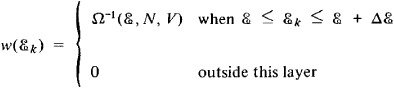Microcanonical Ensemble

Also found in: Wikipedia.

microcanonical ensemble

[¦mī·krō·kə′nän·ə·kəl än′säm·bəl]
(statistical mechanics)
A collection of systems describing a single isolated system of specified energy; its members are uniformly distributed over a part of phase space whose energies lie within an infinitesimal range.
McGraw-Hill Dictionary of Scientific & Technical Terms, 6E, Copyright © 2003 by The McGraw-Hill Companies, Inc.
The following article is from The Great Soviet Encyclopedia (1979). It might be outdated or ideologically biased.

Microcanonical Ensemble

a statistical ensemble of isolated, macroscopic systems (systems that do not exchange energy with surrounding bodies) in a constant volume with a constant number of particles. The energy of systems of microcanonical ensembles has a strictly constant value. The concept of a microcanonical ensemble, introduced by J. W. Gibbs in 1901, is an idealization since, in reality, completely isolated systems do not exist.

In classical statistics, a statistical ensemble is characterized by the distribution function f(qi, pi), which is a function of the coordinates q\ and momenta /?, of all the particles of the system. This function determines the probability for a microscopic state of the system, that is, the probability that the coordinates and momenta of the system’s particles have given values. All microscopic states corresponding to a given energy are equally probable according to the Gibbs microcanonical distribution. (A given energy of the system can be realized for different coordinates and momenta of the system’s particles.)

If we denote by H(qi pi) the system’s energy as a function of the coordinates and momenta (the Hamiltonian function) and if we let & be a given value of the energy, then

f(qi,pi) = A δ { H(qi,pi)—ℰ}

where δ is the Dirac delta function and the constant A is determined by a normalization condition (the total probability for the system to be in all possible states, which may be determined by the integral of f(qi,pi) over all the qi and pi, is equal to unity) and depends on the volume and energy of the system.

In quantum statistics we consider an ensemble of energetically isolated quantum systems with constant volume V and total number of particles N, where the systems have identical energy ℰ with an accuracy to Δℰ ≪ ℰ. It is assumed that all the quantum-mechanical states with energies S* in the layer from ℰ to ℰ + Δℰ are equally probable. Such a probability distribution w of the states of the system, given asis said to be a microcanonical distribution. Here H (S, N, V) is a statistical weight that can be determined from the normalization condition Σkw(ℰ) = 1 and is equal to the number of quantum states in the layer ℰ, ℰ + Δℰ. The quantity AS is usually chosen to be small but finite since an exact determination of energy in quantum mechanics would require, in accordance with the uncertainty relation between energy and time, an infinite observation time. A microcanonical ensemble, however, is not very sensitive to the choice of the width of the energy layer Δℰ—as long as this width is significantly less than the total energy of the system. Therefore, an ensemble of completely isolated systems when Δℰ—0 may also be considered in quantum statistics.

Using the statistical weight Ω(ℰ, N, V), it is possible to calculate various thermodynamic potentials and the entropy S of the system:Δℰ

S = k In Ω(ℰ, N,V)

where k is the Boltzmann constant. Since the entropy of a system is proportional to the number of particles N, the statistical weight has the order of magnitude of an exponential function in N and is very large for the macroscopic systems under consideration.

The microcanonical ensemble is inconvenient for practical application since in order to calculate the statistical weight it is necessary to find the distribution of quantum levels of a system that consists of a large number of particles, which is an extremely complex problem. Instead of considering energetically isolated systems, it is more convenient to consider systems in thermal contact with an environment whose temperature is assumed to be constant (a thermostat) and to use a Gibbs canonical distribution. It is also more convenient to consider the system to be in thermal contact or physical contact with a thermostat (that is, we consider systems which may exchange particles and energy with the thermostat) and to use the Gibbs grand canonical distribution. Gibbs proved that a small part of a microcanonical ensemble is canonically distributed (Gibbs theorem). This theorem may be considered to be the foundation of the Gibbs canonical distribution if a microcanonical distribution is taken as the fundamental postulate of statistical physics.

G. IA. MIAKISHEV and D. N. ZUBAREV.